Published

# Digital thermometer by using Lm35 with Arduino and Iot bolt

Hii..I''am gonna show you my project and the name of my project is digital thermometer by using lm35 with arduino and lcd(16*2).

BeginnerFull instructions provided476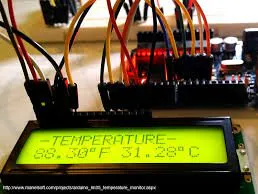## Things used in this project

### Hardware components

 LM35DZ/NOPB
×1Arduino UNO & Genuino UNO
×1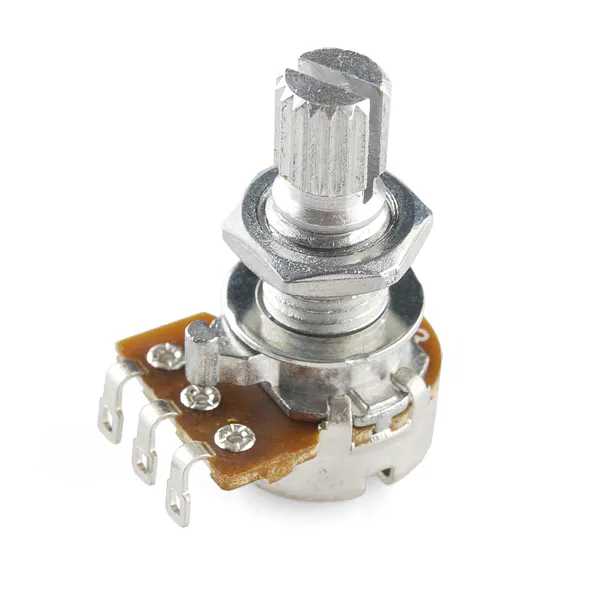Rotary potentiometer (generic)
×1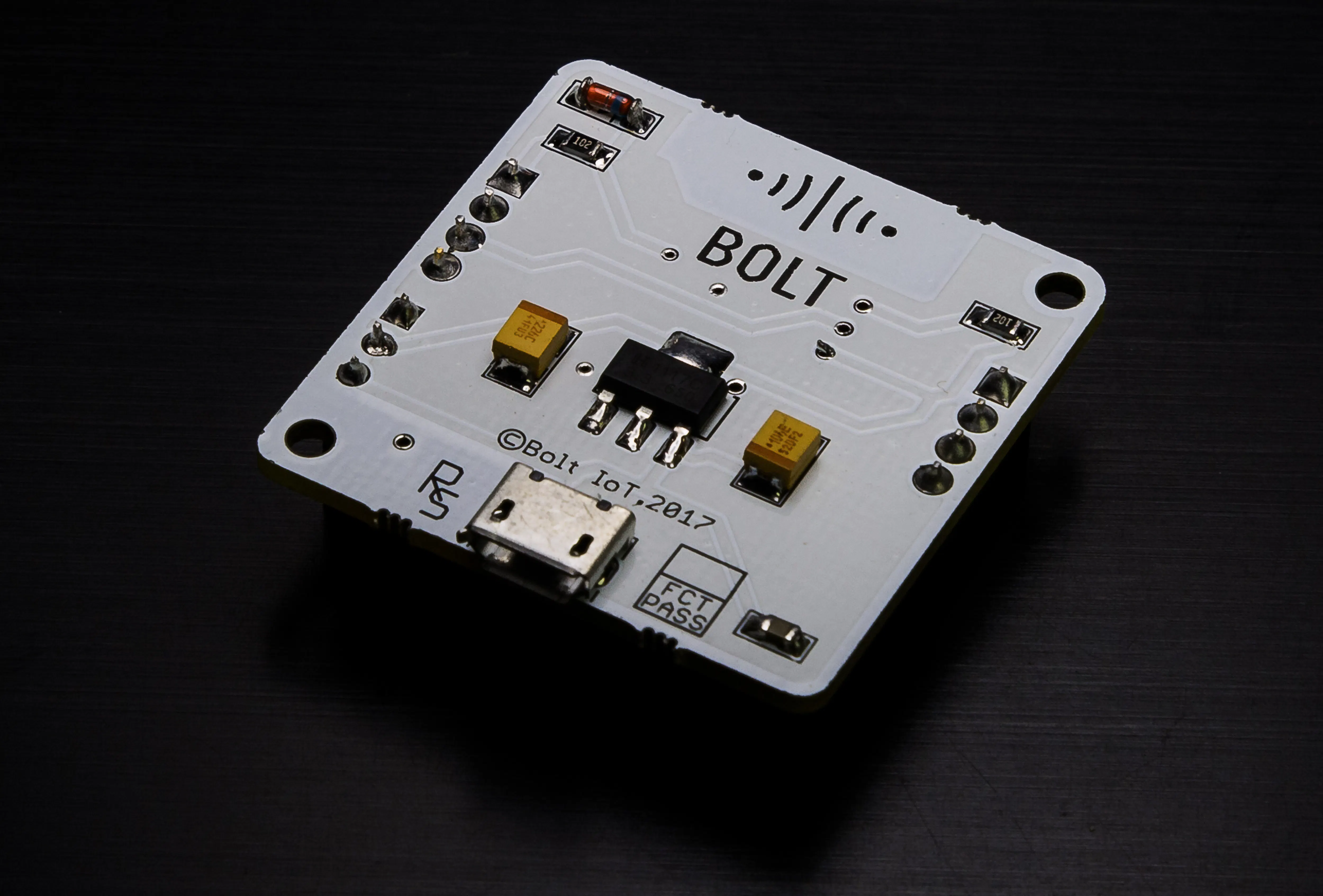Bolt IoT Bolt WiFi Module
×1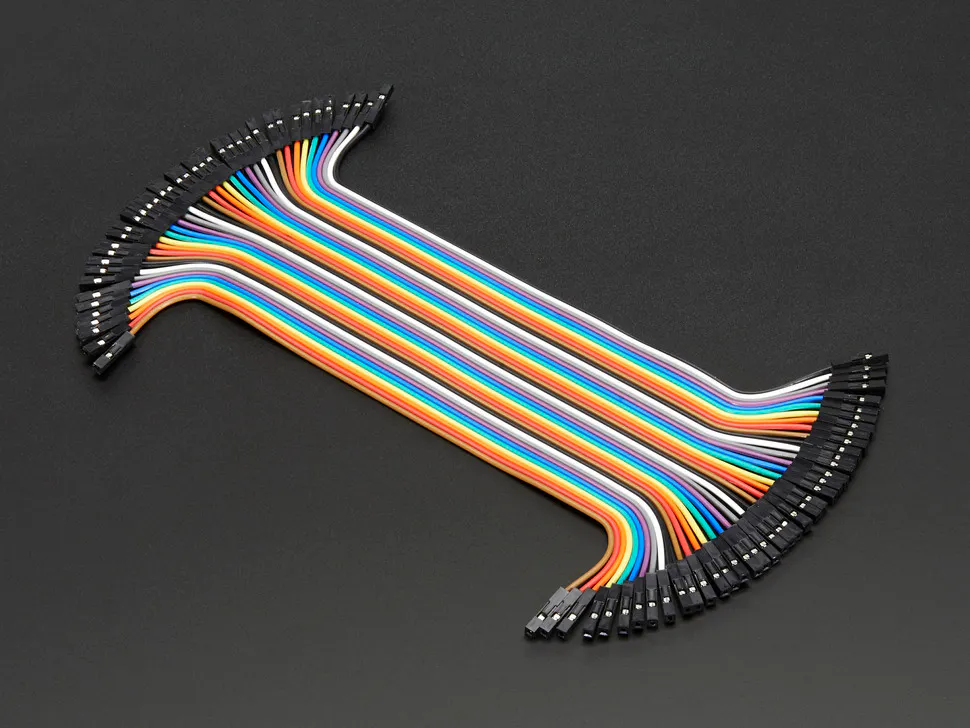Female/Female Jumper Wires
×1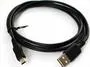USB-A to Mini-USB Cable
×1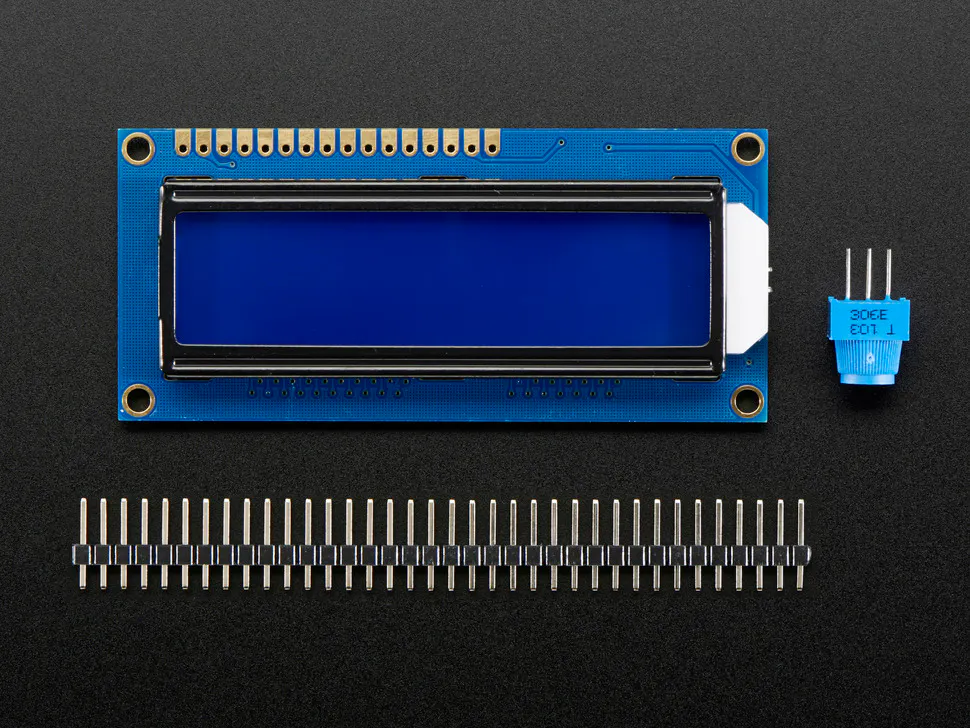Adafruit Standard LCD - 16x2 White on Blue
×1

### Software apps and online servicesBolt IoT Bolt CloudArduino IDE

## Schematics

### digital thermometer using lm35

so, as you can see that i have done all connections between arduino lcd,lm35,wifi module.once you done with all connection then write the program in arduino you will get temp.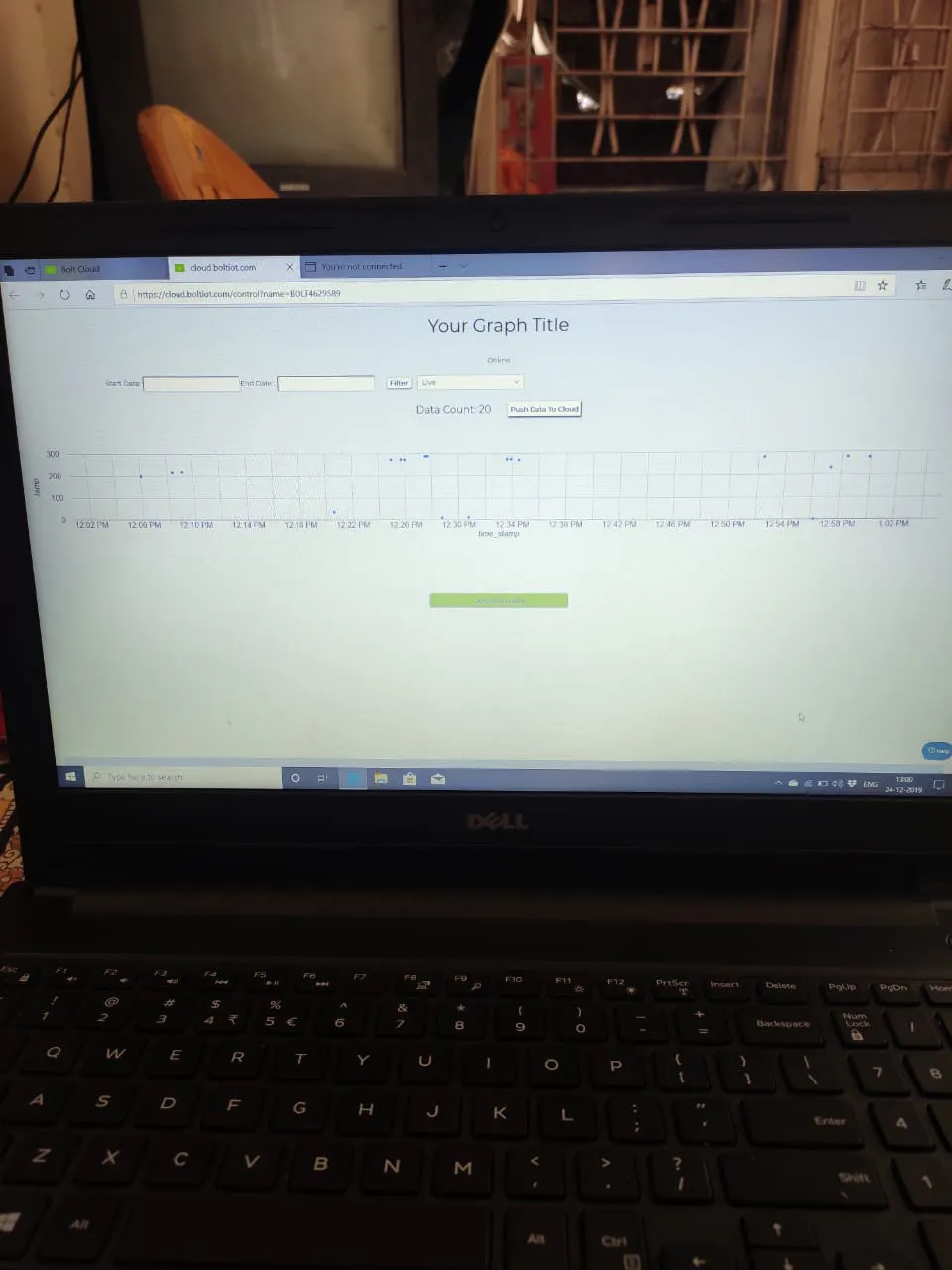### temp.on lcd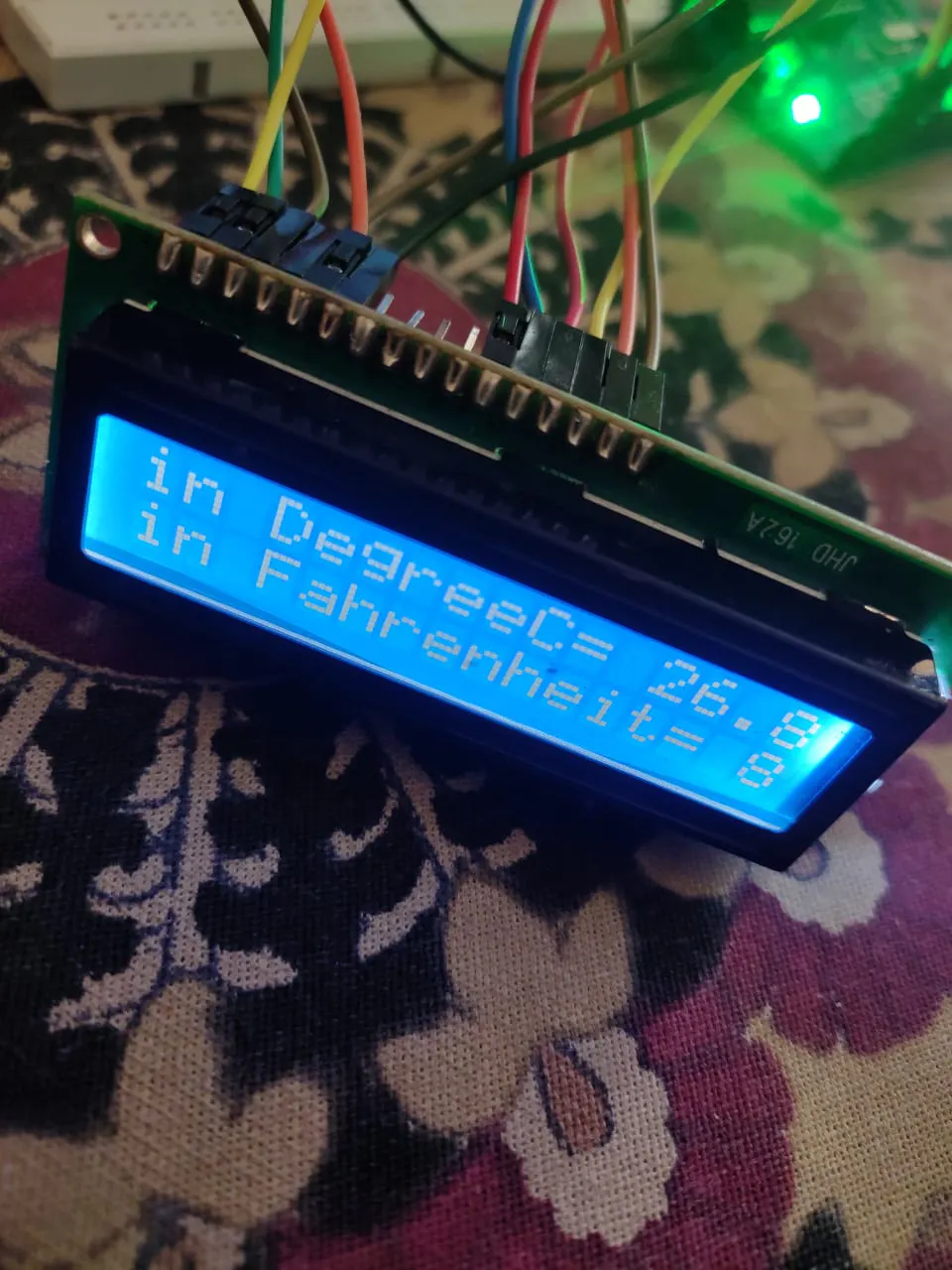### all connection with arduino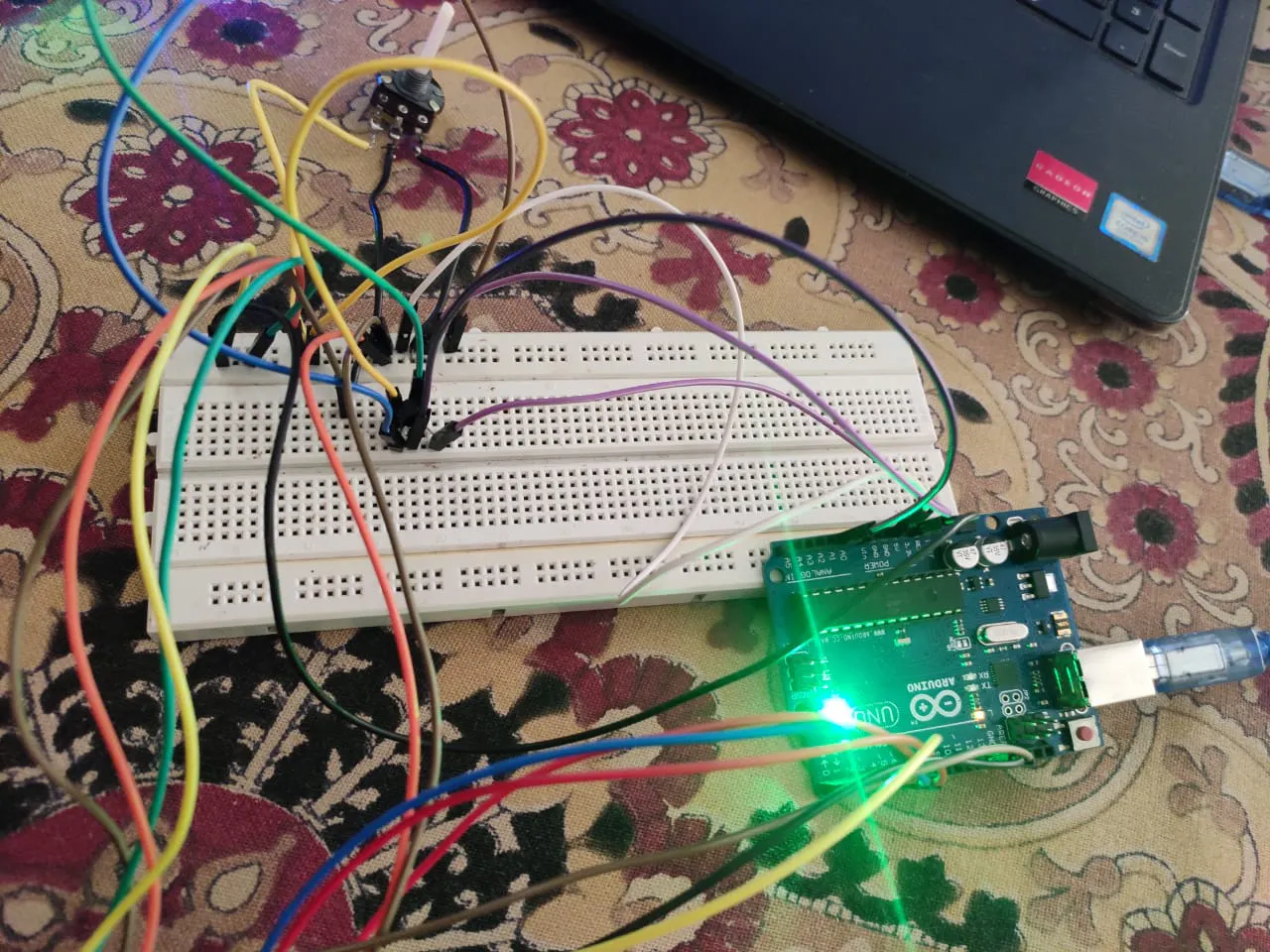### line graph## Code

### the program

Arduino
```#include<LiquidCrystal.h>
LiquidCrystal lcd(12, 11, 5, 4, 3, 2);
const int sensor=A1; // Assigning analog pin A1 to variable 'sensor'
float tempc;  //variable to store temperature in degree Celsius
float tempf;  //variable to store temperature in Fahreinheit
float vout;  //temporary variable to hold sensor reading
void setup()
{
pinMode(sensor,INPUT); // Configuring pin A1 as input
Serial.begin(9600);
lcd.begin(16,2);
delay(500);
}
void loop()
{
vout=(vout*500)/1023;
tempc=vout; // Storing value in Degree Celsius
tempf=(vout*1.8)+32; // Converting to Fahrenheit
lcd.setCursor(0,0);
lcd.print("in DegreeC= ");
lcd.print(tempc);
lcd.setCursor(0,1);
lcd.print("in Fahrenheit=");
lcd.print(tempf);
delay(1000); //Delay of 1 second for ease of viewing in serial monitor
}
```

### the program

Arduino
so, write the program to check temperature in celsius as well as farenheite for that firstly make all the connection between arduino and lcd then put the lm35 and write the program which is given below.
```#include<LiquidCrystal.h>
LiquidCrystal lcd(12, 11, 5, 4, 3, 2);
const int sensor=A1; // Assigning analog pin A1 to variable 'sensor'
float tempc;  //variable to store temperature in degree Celsius
float tempf;  //variable to store temperature in Fahreinheit
float vout;  //temporary variable to hold sensor reading
void setup()
{
pinMode(sensor,INPUT); // Configuring pin A1 as input
Serial.begin(9600);
lcd.begin(16,2);
delay(500);
}
void loop()
{
vout=(vout*500)/1023;
tempc=vout; // Storing value in Degree Celsius
tempf=(vout*1.8)+32; // Converting to Fahrenheit
lcd.setCursor(0,0);
lcd.print("in DegreeC= ");
lcd.print(tempc);
lcd.setCursor(0,1);
lcd.print("in Fahrenheit=");
lcd.print(tempf);
delay(1000); //Delay of 1 second for ease of viewing in serial monitor
}
```

## Credits

### Vallabh deshpande

2 projects • 1 follower